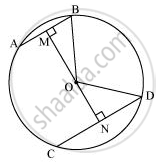# Two chords AB and CD of lengths 5 cm 11cm respectively of a circle are parallel to each other and are on opposite sides of its centre. If the distance between AB and CD is 6 cm, find the radius of the circle. - Mathematics

Two chords AB and CD of lengths 5 cm 11cm respectively of a circle are parallel to each other and are on opposite sides of its centre. If the distance between AB and CD is 6 cm, find the radius of the circle.

#### Solution

Draw OM ⊥ AB and ON ⊥ CD. Join OB and OD.BM = AB/2 = 5/2 (Perpendicular from the centre bisects the chord)

ND = CD/2 = 11/2

Let ON be x. Therefore, OM will be 6− x.

In ΔMOB,

OM2 + MB2 = OB2

(6 - x)2 + (5/2)2 = OB2

36 + x2 - 12x + 25/4 = OB2                 ........(1)

In ΔNOD,

ON2 + ND2 = OD2

x2 + (11/2)2 = OD2

x2 + 121/4 = OD2                          .........(2)

We have OB = OD (Radii of the same circle)

Therefore, from equation (1) and (2),

36+x^2-12x+25/4=x^2+121/4

12x=36+24/4-121/4

=(144+25-121)/4

=48/4

= 12

x = 1

From equation (2),

(1)^2+(121/4)=OD^2

OD^2 = 1+121/4=125/4

OD=5/2sqrt5

Therefore, the radius of the circle is  5/2sqrt5" cm."

Is there an error in this question or solution?

#### APPEARS IN

NCERT Class 9 Maths
Chapter 10 Circles
Exercise 10.6 | Q 2 | Page 186